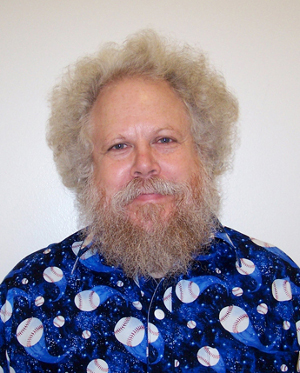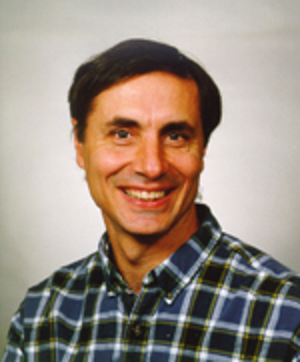# East Coast Computer Algebra Day 2017

## Invited Speakers

### John P. D'Angelo

John P. De'Angelo (University of Illonois, USA)
Title: Take it to the limit, one more time.
Abstract: We discuss theory, examples, and coding techniques used in computing limits of functions of several variables.

### James H. Davenport

James H. Davenport (University of Bath, U.K.)
Title: The ‘doubly-exponential’ problem in equation/inequality solving.
Abstract: It is well-known that the degrees of Gr\”obner Bases are doubly exponential in the number of variables [MayrMeyer1982]. Similarly, real quantifier elimination can be doubly exponential in the number of variables [DavenportHeintz1988]. Recent results show that Gr\”obner bases are only doubly exponential in the dimension, not the actual number of variables. In joint work with Matthew England, we have shown that the double exponent in real quantifier elimination can be reduced by the number of equations, provided that the equations (and their iterated resultants) are content-free. We will explain these results, and why the proviso is necessary. We will also introduce the algorithm LazyRealTriangularize [Chen et al., 2013a], which in practice seems to have good behaviour, though there is no theoretical analysis.

### Bruce Reznick

Bruce Reznick (University of Illinois, USA)
Title: Sums of powers of binary quadratic forms.
Abstract: We will talk about sums of powers of binary quadratic forms, concentrating on sextics as sums of two or three quadratic forms.

### Ekaterina Shemyakova

Ekaterina Shemyakova (SUNY, USA)
Title: Differential Operators and Darboux Transformations in Supergeometry
Abstract: In this talk we consider Darboux transformations (DT), which are certain non-group symmetries of linear partial differential equations, in the setting of supergeometry, an area on the borderline between quantum physics and differential geometry.
All our results in this talk will be for the superline (by which we mean a supermanifold of dimension 1|1, i.e. the case of one even and one odd variable). We show that linear partial differential operators on the superline are close by their properties to ordinary differential operators; in particular, we show that every DT on the superline corresponds to an invariant subspace of the source operator and upon a choice of a basis is given by a super-Wronskian formula. On the way we establish important properties of Berezinians (or superdeterminants) which are analogs of textbook formulas for ordinary determinants but seem to be not known in the super case.

### Dan Steffy

Dan Steffy (Oakland University, USA)
Title: Verifying the results of integer programming solvers.
Abstract: Mixed-integer linear programming is an optimization paradigm with a wide range of applications. Although most software to solve these problems is based on numerical computation, exact and provably correct results are desirable for several applications. This talk will describe a system developed for the exact verification of the results of integer programming solvers. We will describe how the solution algorithms can adapted to output a proof of their conclusions using a limited number of simple inference rules and discuss computational experience with generating and verifying such proofs of correctness. This talk is based on joint work with Kevin K.H. Cheung and Ambros Gleixner.